Smartick is a fun way to learn math!Nov16

# Multiplication Tables and Tricks to Learn Them

In today’s post, we are going to review the multiplication tables (times tables) with a trick so they don’t seem so long.

Are you ready? Let’s get started!

Index

## How Can We Learn Multiplication Tables Quickly?

Have you noticed that all multiplication tables repeat numbers?

Here’s an example:

• In the 1 times table, we have 1 x 2 which is equal to 2.
• If we take the 2 times table we see that 2 x 1 is also equal to 2.
• Therefore, if we know that 1 x 2 = 2, we don’t need to learn that 2 x 1 = 2 because we know and have learned this with the 1 times table.
• We can say that 1 x 2 is the same as 2 x 1.

This occurs because of the communitive property of multiplication: the order of the factors does not change the value of the product. This post explains the properties of multiplication in case you don’t remember.

That’s why we are going to look at the shorter multiplication tables today, avoiding all the repeating multiplication tables, that we don’t need to learn because we already know them!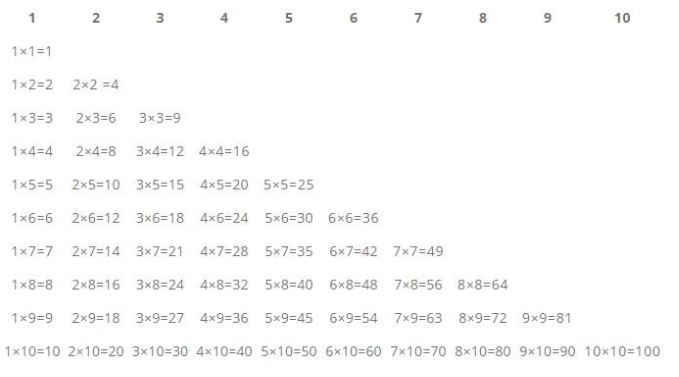As you can see, there are fewer multiplications to learn than originally thought.

In the following images you can see the complete tables, take a look.

### 1 Times Table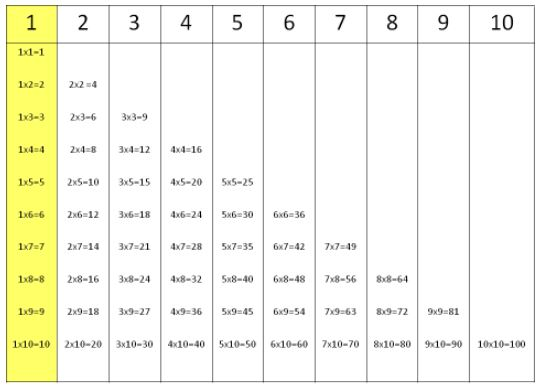### 2 Times Table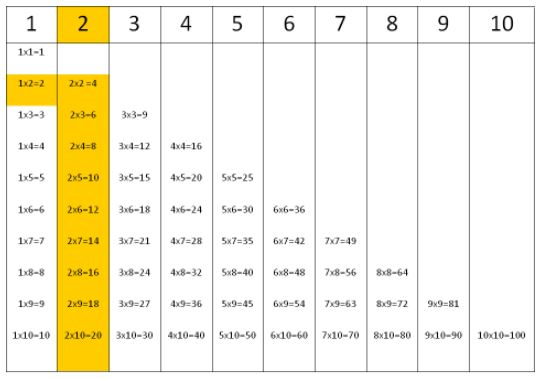### 3 Times Table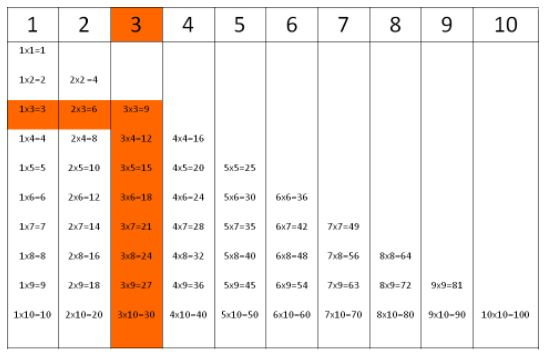### 4 Times Table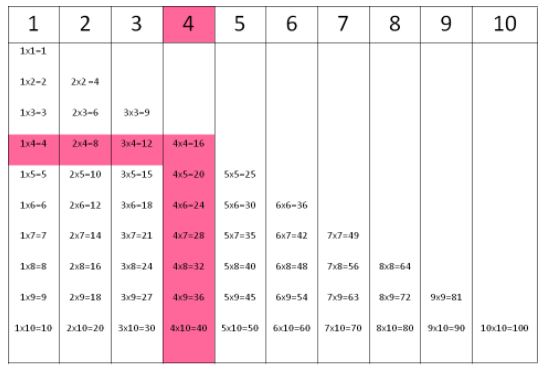### 5 Times Table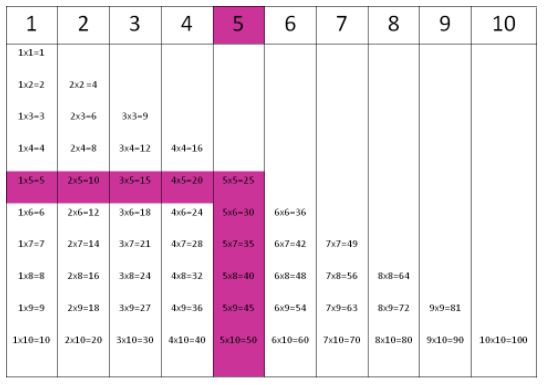### 6 Times Table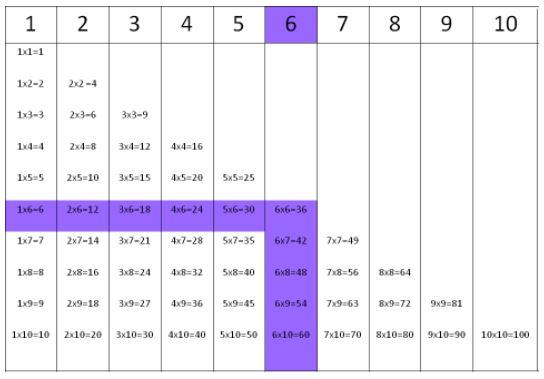### 7 Times Table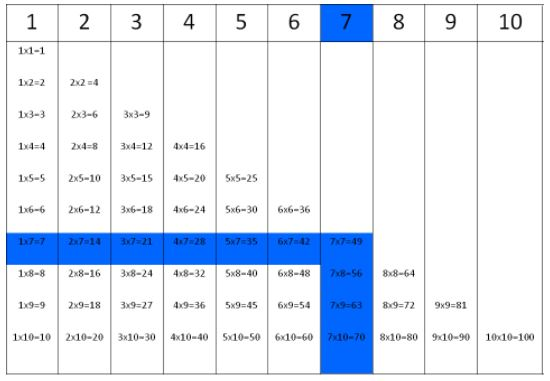### 8 Times Table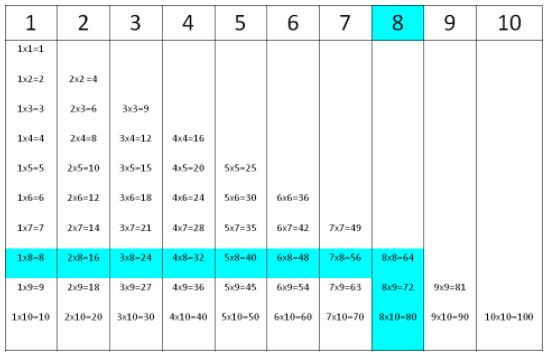### 9 Times Table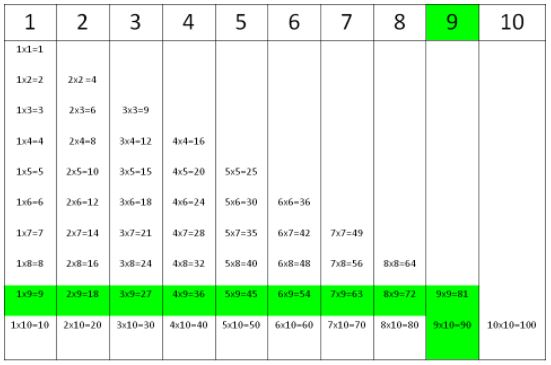### 10 Times Table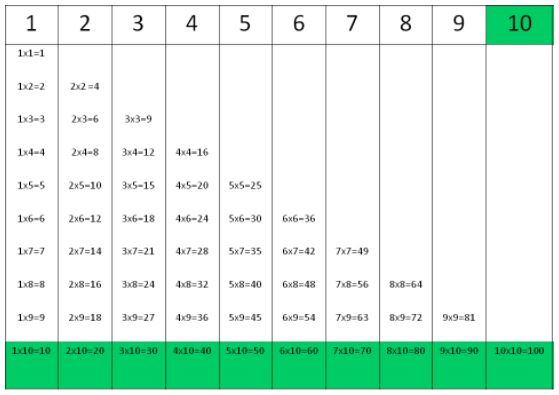What do you think of this trick to learn multiplication tables?

## Video: Commutative Property of Multiplication

If you would like, you can watch the following video where we explain how the commutative property of multiplication works.

This is a video of one of our interactive tutorials. Although it is not interactive in this post, you can still watch it as many times as you need to and share it with friends. If you would like to access our interactive tutorials, register with Smartick! The online method that helps children ages 4 to 14 learn and practice mathematics.

We’re sure you’ll be able to understand this multiplication trick with the help of this video.

## Exercises for Multiplication Tables

Now that we’ve seen the trick to learn multiplication tables faster, would you like to practice some?

Take a look at our post about multiplication tables and you’ll find tons of activities that children do during their Smartick sessions.

Give it a try!

## How to Teach Multiplication Tables

One of the fundamental tricks for teaching multiplication tables is the one that we are explaining in this post: the communitive property of multiplication which means that the order of factors does not change the product.

Therefore, if you know 4 x 6, you will also know the result of 6 x 4.

In addition to this trick, we will give you some basic instructions to teach multiplication tables.

The 1 times table is the easiest because the product is always the same as the number that we are multiplying by.

For example:

1 × 1 = 1

1 × 2 = 2

1 × 3 = 3

1 × 4 = 4

### Continue with 2

The 2 times table goes through even numbers, that is, learning the 2 times tables is done by learning to count by 2. Think about it, the result of multiplying by 2 is always the double of some number, an even number. Take advantage of this, in the 2 times tables the product of a multiplication problem is double the number you are multiplying by.

In other words:

2 × 1 = 2

2 × 2 = 4

2 × 3 = 6

2 × 4 = 8

### The 4 Times Table is Double the 2 Times Table

4 × 1 = 4

4 × 2 = 8

4 × 3 = 12

4 × 4 = 16

Like we had said about the 2 times tables, learning to quickly calculate doubles of any number is going to open doors, the times tables for 4, 6 (which is the double of 3), 8 (the double of 4)…all of this is much easier if you know how to accurately calculate doubles.

### The 5 Times Table is Very Easy: Adding 5 by 5

The 5 times table is also very easy to learn. The only thing you need to do is add 5 by 5.

For example:

5 × 1 = 5

5 × 2 = 10

5 × 3 = 15

5 × 4 = 20

Do you see it?

### An Old Trick for the 9 Times Tables

All of the tables can be built by adding the multiplicand however many times as the multiplier states so that adding 9 is like adding 10 and subtracting 1. There is a strange trick for the 9 times table, begin by putting the number of the tens (blue) in the product in increasing order (0 to 9) and continue by adding the digits to the ones (red) in the reverse order (9 to 0).

9 × 1 = 09

9 × 2 = 18

9 × 3 = 27

9 × 4 = 36

9 × 5 = 45

9 × 6 = 54

9 × 7 = 63

9 × 8 = 72

9 × 9 = 81

9 × 10 = 90

## Multiplication Tables to Print

In this post, you can download and print the times tables in order to practice them and commit them to memory.

If you liked these tricks to learn and teach multiplication tables, log on to Smartick to register and try it for free! Discover all of our content that can help your children learn mathematics adapted to their level.